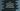# endl in C++ and why we use it instead of newline## endl in C++ :

endl stands for end line in C++. You can use this operator to insert a new line at the end of a line. It puts one new line and flushes the stream. Let me show you one small example :

``````#include <iostream>

int main()
{
std::cout << "First line..." << std::endl;
std::cout << "Second line..." << std::endl;
std::cout << "Third line..." << std::endl
<< "Fourth line..." << std::endl;
}``````

It will print the below output :

``````First line...
Second line...
Third line...
Fourth line...``````

You can also use using namespace std if you don’t want to use std each time.

``````#include <iostream>
using namespace std;

int main()
{
cout << "First line..." << endl;
cout << "Second line..." << endl;
cout << "Third line..." << endl
<< "Fourth line..." << endl;
}``````

It will print the same output.

### Why we use endl instead of newline character :

Newline character ‘\n’ is another way to add a newline. Actually, endl appends ‘\n’ and calls flush() to flushes the stream.

So,

``cout << "Hello"<< endl;``

is equivalent to :

``````cout << "Hello"<< '\n';
cout.flush();``````